×

Search anything:

# Largest Independent Set in Binary Tree

#### Problems on Binary Tree Algorithms Data Structures Dynamic Programming (DP)Reading time: 30 minutes | Coding time: 10 minutes

Given a Binary Tree, we have to find the size of Largest Independent Set (LIS) in it. A subset of all tree nodes is an independent set if there is no edge between any two nodes of the subset.

For example, consider the following binary tree. The largest independent set(LIS) is {1, 4, 8, 7, 5} and size of the LIS is 5.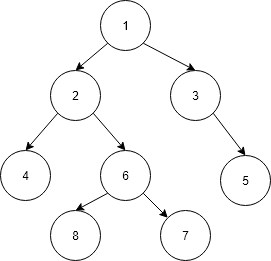A Dynamic Programming solution solves a given problem using solutions of subproblems in bottom up manner. Can the given problem be solved using solutions to subproblems? If yes, then what are the subproblems? Can we find largest independent set size (LISS) for a node X if we know LISS for all descendants of X? If a node is considered as part of LIS, then its children cannot be part of LIS, but its grandchildren can be. Following is optimal substructure property.

Let LISS(X) indicates size of largest independent set of a tree with root X.

``````LISS(X) = largest independent set with node X as root
``````

The recursive structure is as follows:

``````LISS(X) = MAX { (1 + sum of LISS for all grandchildren of X),
(sum of LISS for all children of X) }
``````

The idea is simple, there are two possibilities for every node X:

• either X is a member of the set
• X is not a member.

The implications are:

• If X is a member, then the value of LISS(X) is 1 plus LISS of all grandchildren.
• If X is not a member, then the value is sum of LISS of all children.

Following is recursive implementation that simply follows the recursive structure mentioned above.

``````// A naive recursive implementation of
// Largest Independent Set problem
#include <bits/stdc++.h>
using namespace std;

// A utility function to find
// max of two integers
int max(int x, int y)
{
return (x > y) ? x : y;
}

/* A binary tree node has data,
pointer to left child and a
pointer to right child */
class node
{
public:
int data;
node *left, *right;
};

// The function returns size of the
// largest independent set in a given
// binary tree
int LISS(node *root)
{
if (root == NULL)
return 0;

// Calculate size excluding the current node
int size_excl = LISS(root->left) +
LISS(root->right);

// Calculate size including the current node
int size_incl = 1;
if (root->left)
size_incl += LISS(root->left->left) +
LISS(root->left->right);
if (root->right)
size_incl += LISS(root->right->left) +
LISS(root->right->right);

// Return the maximum of two sizes
return max(size_incl, size_excl);
}

// A utility function to create a node
node* newNode( int data )
{
node* temp = new node();
temp->data = data;
temp->left = temp->right = NULL;
return temp;
}

// Driver Code
int main()
{
// Let us construct the tree
// given in the above diagram
node *root = newNode(1);
root->left = newNode(2);
root->left->left = newNode(4);
root->left->right = newNode(6);
root->left->right->right = newNode(7);
root->left->right->left = newNode(8);
root->right = newNode(3);
root->right->right = newNode(5);

cout << "Size of the Largest"
<< " Independent Set is "
<< LISS(root);

return 0;
}
``````

Output:

``````Size of the Largest Independent Set is 5
``````

Time complexity of the above naive recursive approach is exponential.

It should be noted that the above function computes the same subproblems again and again. Since same suproblems are called again, this problem has Overlapping Subprolems property. So LISS problem has both properties of a dynamic programming problem. Like other typical Dynamic Programming (DP) problems, recomputations of same subproblems can be avoided by storing the solutions to subproblems and solving problems in bottom up manner.

This Dynamic programming problem can be solved by augmented tree.

The augmented tree conains nodes having:

• data
• left child
• right child
• liss (an extra field)

## Dynamic Programming Algorithm

The basic idea is as follows:

• the size of largest independent set excluding root is the size for the left tree + right tree. This is because at this point it might not be clear that including root is compatible or not.
``````size excluding root = size(root->left) + size(root->right)
``````
• If we include the root, the minimum size is 1 and include the size of left node's children and right node's children. This is because left and right nodes cannot be included.
``````size including root = 1 + size(root->left->left) + size(root->left->right) +
size(root->right->left) + size(root->right->right)
``````

Following is the pesudocode:

``````Begin.
Create a structure n to declare data d, a left child pointer l and a right child pointer r.
Call a function max() to return maximum between two integers. Create a function LIS() to return the
size of the largest independent set in a given binary tree.
Calculate size excluding the current node
int size_excl = LIS(root->l) + LIS(root->r)
Calculate size including the current node
int size_incl = 1;
if (root->l)
size_incl += LIS(root->l->l) + LIS(root->l->r)
if (root->right)
size_incl += LIS(root->r->l) + LIS(root->r->r)
Return the maximum of two sizes
Create a function to create newnode.
End.
``````

Following are implementation of Dynamic Programming based solution. In the following solution, an additional field ‘liss’ is added to tree nodes. The initial value of ‘liss’ is set as 0 for all nodes. The recursive function LISS() calculates ‘liss’ for a node only if it is not already set.

``````/* Dynamic programming based program
for Largest Independent Set problem */
#include <bits/stdc++.h>
using namespace std;

// A utility function to find max of two integers
int max(int x, int y) { return (x > y)? x: y; }

/* A binary tree node has data, pointer
to left child and a pointer to
right child */
class node
{
public:
int data;
int liss;
node *left, *right;
};

// A memoization function returns size
// of the largest independent set in
// a given binary tree
int LISS(node *root)
{
if (root == NULL)
return 0;

if (root->liss)
return root->liss;

if (root->left == NULL && root->right == NULL)
return (root->liss = 1);

// Calculate size excluding the current node
int liss_excl = LISS(root->left) + LISS(root->right);

// Calculate size including the current node
int liss_incl = 1;
if (root->left)
liss_incl += LISS(root->left->left) + LISS(root->left->right);
if (root->right)
liss_incl += LISS(root->right->left) + LISS(root->right->right);

// Maximum of two sizes is LISS, store it for future uses.
root->liss = max(liss_incl, liss_excl);

return root->liss;
}

// A utility function to create a node
node* newNode(int data)
{
node* temp = new node();
temp->data = data;
temp->left = temp->right = NULL;
temp->liss = 0;
return temp;
}

// Driver code
int main()
{
// Let us construct the tree
// given in the above diagram
node *root = newNode(1);
root->left = newNode(2);
root->left->left = newNode(4);
root->left->right = newNode(6);
root->left->right->right = newNode(7);
root->left->right->left = newNode(8);
root->right = newNode(3);
root->right->right = newNode(5);

cout << "Size of the Largest Independent Set is " << LISS(root);

return 0;
}
``````

Output:

``````Size of the Largest Independent Set is 5
``````

## Explanation

• The idea is to maintain two list of alternative nodes i.e. grandchild nodes of nodes.
• First go to the left most node of the tree and make the liss field of that node is equal to one.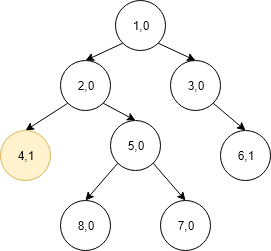• Then go to the right most node and the liss field of that node equal to one and liss_excl = 2.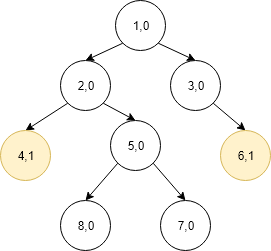• Now liss_incl = 1 and now go to the another leaf node i.e 8 and make the liss of that equal to one and.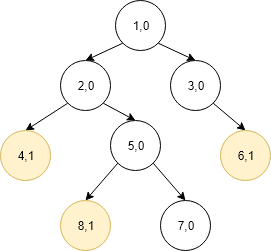• Then go the right leaf node and i.e. 7 and make liss of that equal to one and liss_incl=3.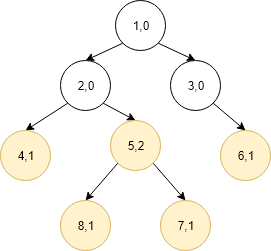• Then go the parent and make its liss equal to liss_excl and liss_incl.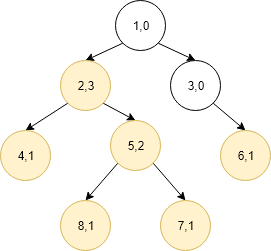• Now assign the value of liss_incl to the parent.• Now go the right of the root and assign its liss equal to one and liss_incl = 5 and assign root node liss equal to liss_incl.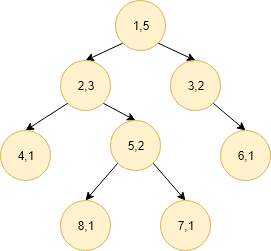Time Complexity: O(n) where n is the number of nodes in given Binary tree.#### Parth Maniyar

Competitive Programmer | Intern at OpenGenus | Bachelor of Technology (2017 to 2021) in Information Technology at Ahmedabad University

Largest Independent Set in Binary Tree